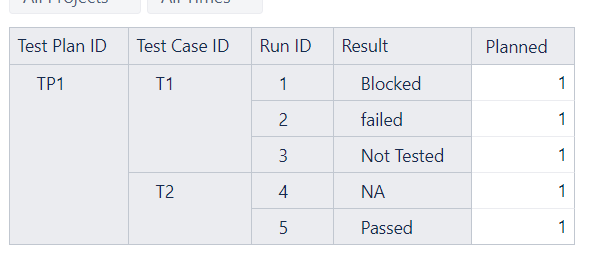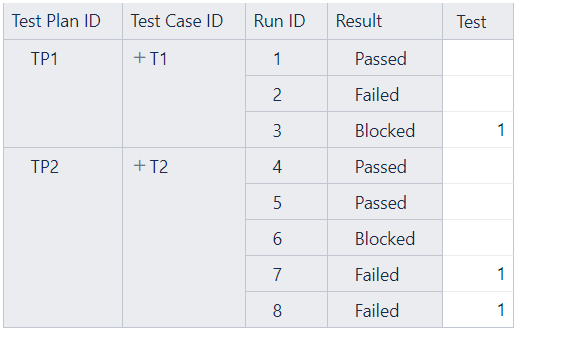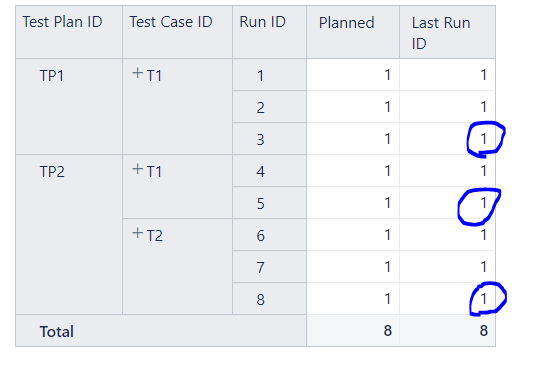# Creating a new calculated measure for a maximum value in a dimension

Hi,

I have been trying to do this but missing something. Here I want a new column “Overall Result” which gives the result of maximum run id for each test case.
For example for Test case T1, new column should give “Not Tested” as the Result for max Run ID (3) is Not Tested.
For Test case T2 it should give me “Passed” as the result for maximum Run ID (5) related to that test case is Passed. I have imported this data from a csv file, so all the headers that you see are the dimensions.
Please let me know if you have any questions.
Thank you.Hi @Pavansai,

I am sorry your question has been without an answer for a long time.

You can create a calculated measure that counts Test Cases only by the last Run results.
The calculations would go through all test runs, order them, and get only the latest Run for each Test Case. Put it in a tuple with measure “Planned”. The expression might look like this:

``````(
Order(
--set of Runs that are related to Plan and Test Case
Filter(
Descendants([Run ID].[Run ID].Members),
([Measures].[Planned],
[Result].DefaultMember) > 0
),
--order Run by name
[Run ID].CurrentMember.Name, BDESC
--get the latest Run
).item(0),
[Measures].[Planned]
)
``````

Now you might want to add the new calculated measure to report columns and remove measure “Planned”. And select to hide empty rows.

More details on calculated measures are here:

Zane / Support@eazyBI.com
Best,

Hi @zane.baranovska ,
This is Brilliant but I think I might have missed one case. If there are multiple values with same results it is pointing to both of them. In this case, how can I get only the latest one. In the attached picture, I want my measures “Test” for Test Plan ID “TP2” to point to Run ID - 8 but it is pointing to both 7 and 8 as it has same result.
Can you please let me know how can I solve this as I need only result of latest Run IDYou might want to remove the dimension “Run ID” from report rows as it is already considered in the calculated measure.

Best,
Zane / Support@eazyBI.com

Hi @zane.baranovska
That worked exactly how I want it to work. Thank you so much. As I’m practising on forming few more calculated measures for my future reference.
Using above calculated measure. I’m trying to point my “Last Run ID” calculated measure to maximum Run ID for each test case under a test plan but I’m missing something.
As per attached picture, here I want to point to 3, 5, and 8 as they are the max Run IDs for test cases under each Test Plan.
I thought that I can easily get this by removing Result.Defaultmember from the above calculated measure given by you but that is not working. Please guide me on this.
Thank you